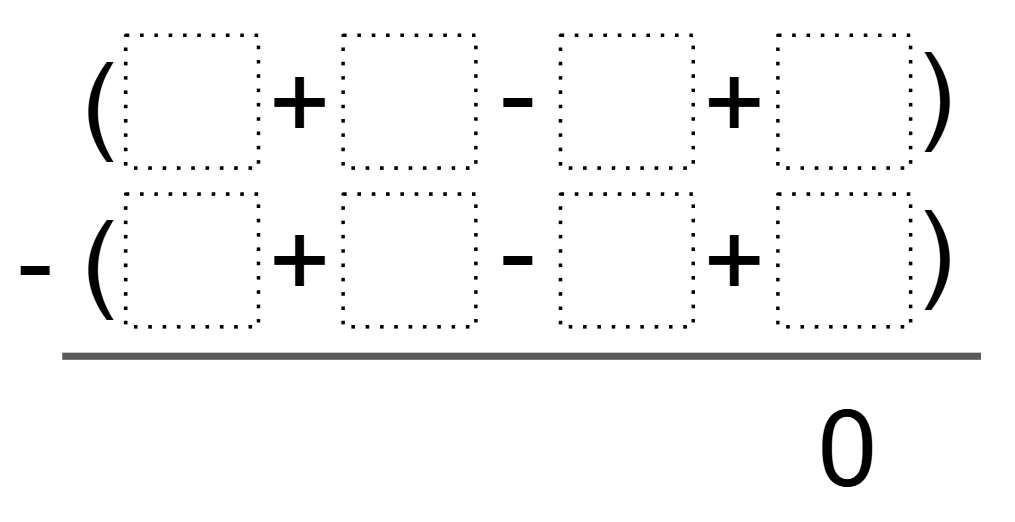# Creating Zero

Directions: Using the digits 1 to 9 at most one time each, fill in the boxes to make the equality true:### Hint

What numbers will produce a difference of zero?

Because of the commutative property, there are many different answer combinations. One possible solution would be: ( 9 + 6 – 5 + 2 ) – ( 8 + 7 – 4 + 1 )

Source: Bryan Anderson

## Two Step Inequality with Fractional Coeffcient

Directions: Using the digits 1 to 7 at most one time each, place a digit …

1.Also, (9+1-8+2) – (7+3-6+4) = 0.

•that would = -4

•you can use those numbers just move them around.

•(9+1-8+2)-(7+3-6=4=0

2.( 9 + 6 – 5 + 2 ) – ( 8 + 7 – 4 + 1 )

3.(9+8-4+3)-(7+6-2+5)=0

4.(9 + 2 – 5 + 6) – (5 + 6 – 2 + 3) = 0

•(9+6-5+2)-(8+7-4+1)=0

5.( 9 + 6 – 5 + 2 ) – ( 8 + 7 – 4 + 1 )

6.( 5 + 2 – 9 + 6 ) – ( 4 + 1 – 8 + 7 )?????

7.(8+9-4+3)-(6+7-2+5)=0

8.Also (4+3-2+8)-(3+4-3+9)=0

9.Because of the commutative property, there are many different answer combinations. One possible solution would be: ( 9 + 6 – 5 + 2 ) – ( 8 + 7 – 4 + 1 )

10.(9+6-5+2)-(8+7-4+1)

11.Person aka kaleya

(9+5-6+2)-(7+8-4+1)

12.( 9 + 6 – 5 + 2 ) – ( 8 + 7 – 4 + 1 )

•( 9 + 6 – 5 + 2 ) – ( 8 + 7 – 4 + 1 )=0

13.(9+1-3+3)-(5+6-10+9)=0

•this one is wrong

•14.(7+8-2+3)-(6+9-1+4)=0

15.( 9 + 6 – 5 + 2 ) – ( 8 + 7 – 4 + 1 )

16.(9+18-6+3)-(7+24-6+7)=0

17.(9+6-5+2)-(8+7-4+1)

18.(5+13-3+7)-(7+12-6+3)

19.(4+5-6+3)-(7+1-6+2)=0

20.Kaylee Crandall

(9+6-5+2)-(8+7-4+1)

21.(9+6-5+2)-(8+7-4+1)

22.(2+(-5)+6+9)-(1+(-4)+7+8)

23.Ahmed Abdullah Syed

( 1 + 9 – 8 + 2 ) – ( 7 + 3 – 4 + 6 )

24.25.( 1 + 9 – 8 – 2 ) – ( 7 + 3 – 4 – 6 )

26.Zachary Forrester

(9+2-1+3)-(4+8-5+6)

27.this is so crzy it is stupid